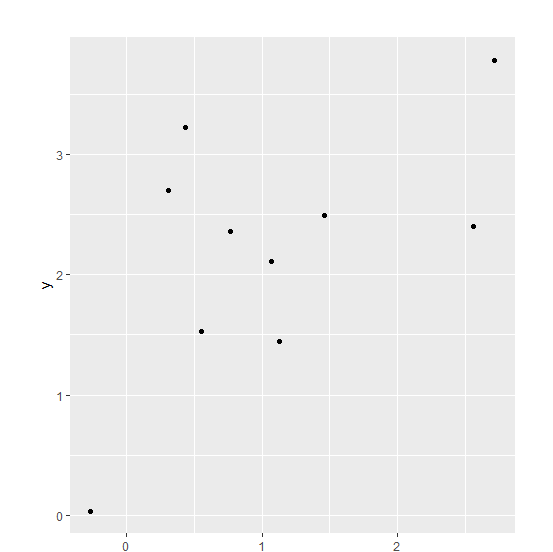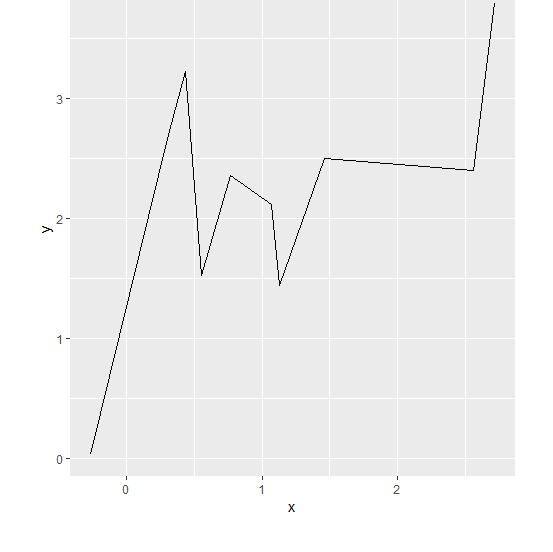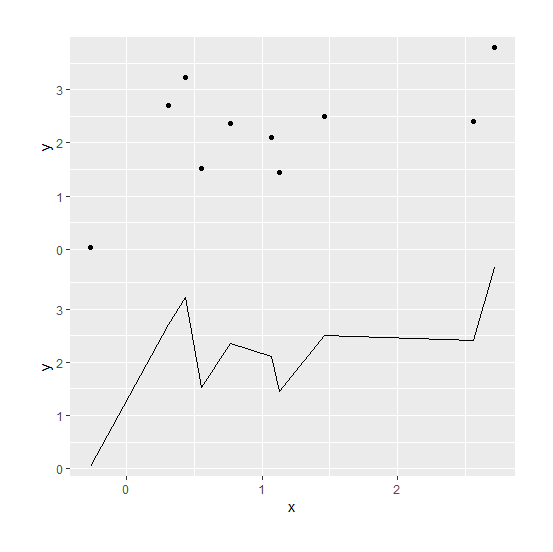# How to reduce the space between two plots that are joined with grid.arrange in R?

When we join or combine plots using grid.arrange the scales of the first plot comes in between as X-axis even if the independent variable in both of the plots is same.

Therefore, we might want to remove the space between the plots while joining to get only one X-axis. This can be done by using theme function.

## Example

Consider the below data frame −

Live Demo

set.seed(123)
x<-rnorm(10,1)
y<-rnorm(10,2)
df<-data.frame(x,y)
df

## Output

      x          y
1 0.4395244 3.22408180
2 0.7698225 2.35981383
3 2.5587083 2.40077145
4 1.0705084 2.11068272
5 1.1292877 1.44415887
6 2.7150650 3.78691314
7 1.4609162 2.49785048
8 -0.2650612 0.03338284
9 0.3131471 2.70135590
10 0.5543380 1.52720859

library(ggplot2)
library(gridExtra)

Creating scatterplot and line chart between x and y with plot.margin to reduce the space between the plot when joined with grid.arrange −

## Example

Plot1<-ggplot(df,aes(x,y))+geom_point()+theme(plot.margin=unit(c(1,1,-0.5,1),"cm"))
Plot1

## OutputPlot2<-ggplot(df,aes(x,y))+geom_line()+theme(plot.margin=unit(c(-0.5,1,1,1),"cm"))
Plot2

## OutputJoining the two plots −

## Example

grid.arrange(Plot1,Plot2)

## Output